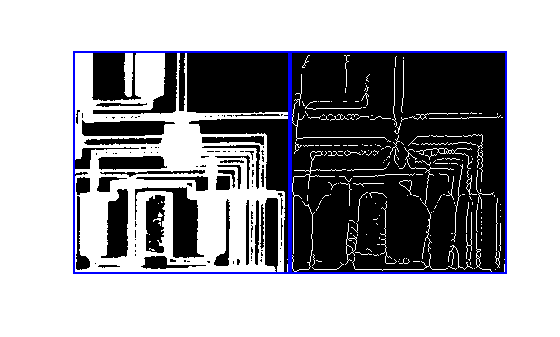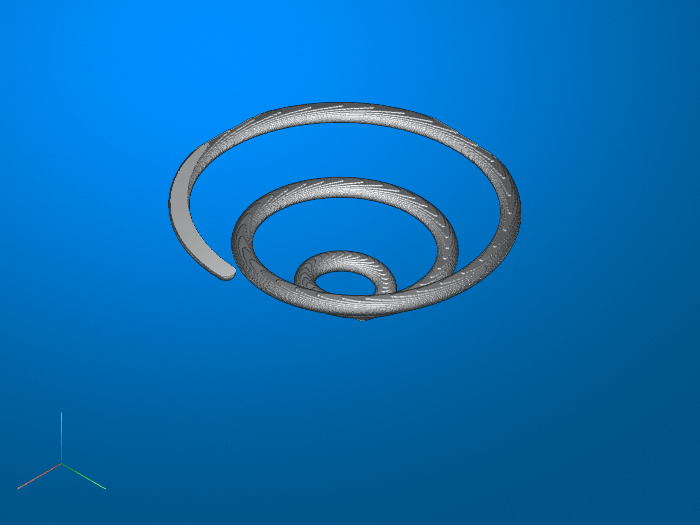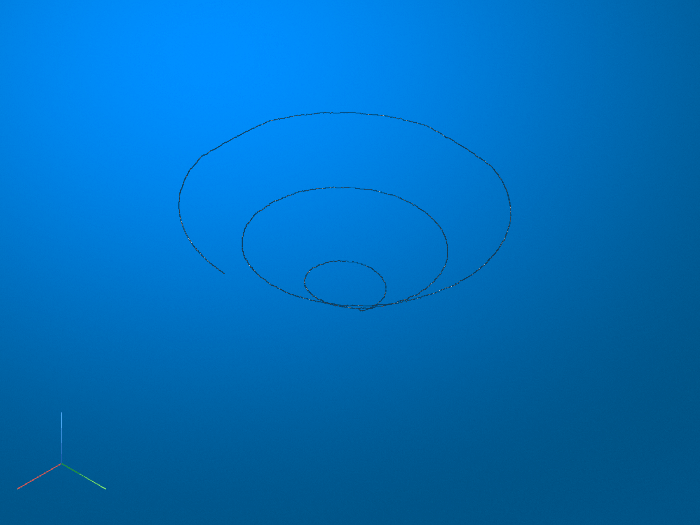# bwskel

Reduce all objects to lines in 2-D binary image or 3-D binary volume

## Syntax

``B = bwskel(A)``
``B = bwskel(V)``
``B = bwskel(___,'MinBranchLength',N)``

## Description

example

````B = bwskel(A)` reduces all objects in the 2-D binary image `A` to 1-pixel wide curved lines, without changing the essential structure of the image. This process, called skeletonization, extracts the centerline while preserving the topology and Euler number (also known as the Euler characteristic) of the objects.```

example

````B = bwskel(V)` returns the skeleton of a 3-D binary volume.```
````B = bwskel(___,'MinBranchLength',N)` specifies the minimum branch length `N` of the skeleton. `bwskel` removes (prunes) all branches shorter than the specified length. `bwskel` calculates the length as the number of pixels in a branch using 8-connectivity for 2-D and 26-connectivity for 3-D.```

## Examples

collapse all

Read a 2-D grayscale image into the workspace. Display the image. Objects of interest are dark threads against a light background.

```I = imread('threads.png'); imshow(I)```Skeletonization requires a binary image in which foreground pixels are `1` (white) and the background is `0` (black). To make the original image suitable for skeletonization, take the complement of the image so that the objects are light and the background is dark. Then, binarize the result.

```Icomplement = imcomplement(I); BW = imbinarize(Icomplement); imshow(BW)```Perform skeletonization of the binary image using `bwskel`.

`out = bwskel(BW);`

Display the skeleton over the original image by using the `labeloverlay` function. The skeleton appears as a 1-pixel wide blue line over the dark threads.

`imshow(labeloverlay(I,out,'Transparency',0))`Prune small spurs that appear on the skeleton and view the result. One short branch is pruned from a thread near the center of the image.

```out2 = bwskel(BW,'MinBranchLength',15); imshow(labeloverlay(I,out2,'Transparency',0))```Read a binary image into the workspace.

`BW1 = imread('circbw.tif');`

Skeletonize objects in the image by using the `bwskel` function.

`BW2 = bwskel(BW1);`

View the original image and the skeletonized image side by side.

`montage({BW1,BW2},'BackgroundColor','blue','BorderSize',5)`Load a volumetric data set into the workspace. The name of the data set is `spiralVol`. Display the volume using `volshow`.

```load spiralVol.mat; volshow(spiralVol);```Convert the `spiralVol` data set to a binary format which is required by the `bwskel` function.

`spiralVolLogical = imbinarize(spiralVol);`

Skeletonize the spiral shape in the data set. Display the skeletonized volume with `volshow`.

`spiralVolSkel = bwskel(spiralVolLogical);`

.## Input Arguments

collapse all

Binary image, specified as a 2-D logical matrix.

Data Types: `logical`

3-D binary volume, specified as a 3-D logical array.

Data Types: `logical`

Minimum branch length, specified as a nonnegative integer. `bwskel` prunes branches shorter than `N`. By default, `bwskel` does not prune branches.

## Output Arguments

collapse all

Skeletonized image or volume, returned as a 2-D logical matrix or 3-D logical array of the same size as the input image or volume.

## Tips

• While both `bwskel` and `bwmorph` can skeletonize 2-D images, you can get different results using `bwmorph` than when using `bwskel`. Because they use different algorithms, the `bwskel` function uses 4-connectivity with 2-D images; `bwmorph` uses 8-connectivity.

• `bwskel` assumes that foreground objects in the binary image are white (logical `true`). If your image has a white background and black objects, then use the complement of your image as the input to `bwskel`. You can compute the complement by using `imcomplement`.

## Algorithms

• The `bwskel` function uses the medial axis transform.

 Ta-Chih Lee, Rangasami L. Kashyap and Chong-Nam Chu. Building skeleton models via 3-D medial surface/axis thinning algorithms. Computer Vision, Graphics, and Image Processing, 56(6):462-478, 1994.

 Kerschnitzki, M, Kollmannsberger, P, Burghammer, M. et al. Architecture of the osteocyte network correlates with bone material quality. Journal of Bone and Mineral Research, 28(8):1837-1845, 2013.